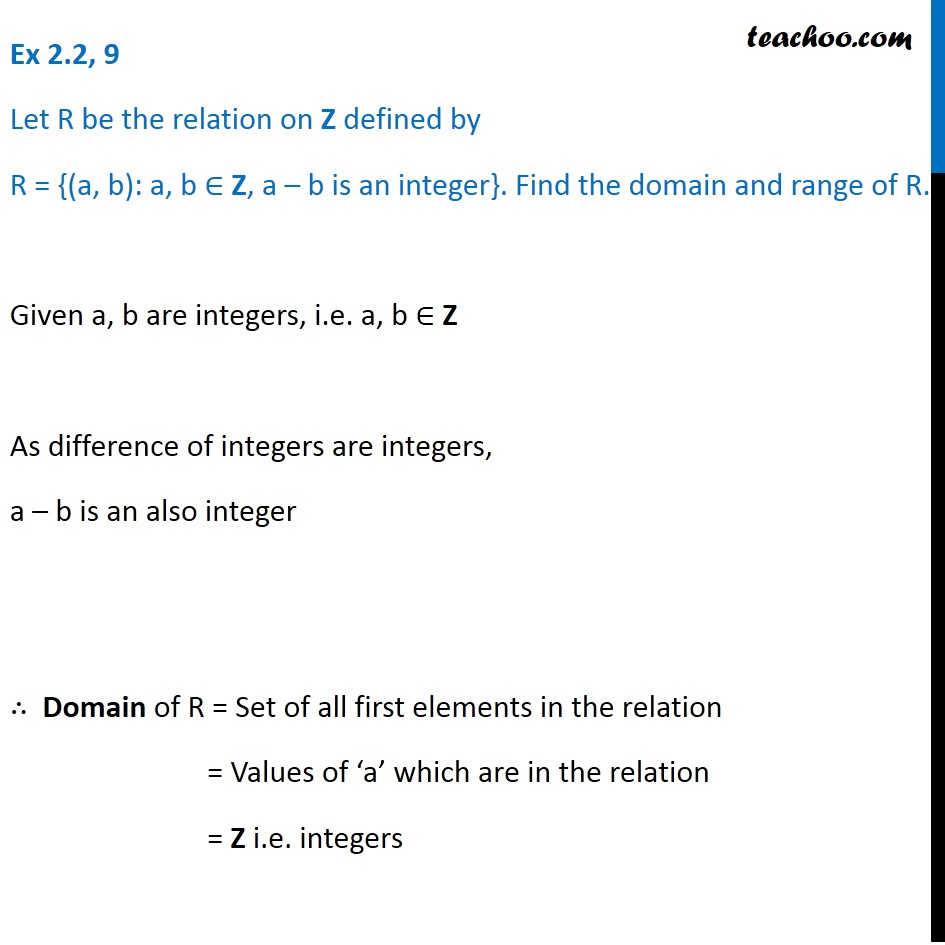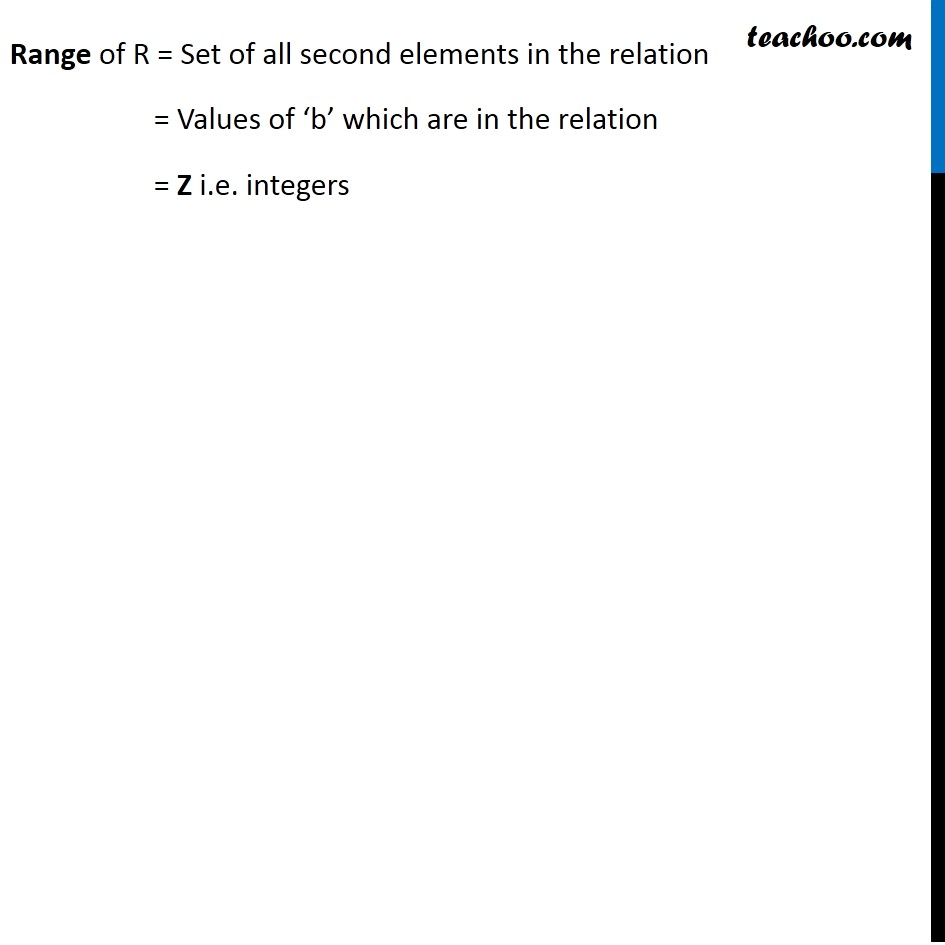Finding Relation - Set-builder form given

Chapter 2 Class 11 Relations and Functions
Concept wiseLearn in your speed, with individual attention - Teachoo Maths 1-on-1 Class

### Transcript

Ex 2.2, 9 Let R be the relation on Z defined by R = {(a, b): a, b ∈ Z, a – b is an integer}. Find the domain and range of R. Given a, b are integers, i.e. a, b ∈ Z As difference of integers are integers, a – b is an also integer ∴ Domain of R = Set of all first elements in the relation = Values of ‘a’ which are in the relation = Z i.e. integers Range of R = Set of all second elements in the relation = Values of ‘b’ which are in the relation = Z i.e. integers